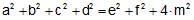# Problem 250. Quadrilateral, sides, diagonals, midpoints, squares

The figure shows a quadrilateral ABCD, E and F midpoints of diagonals AC and BD respectively. If AB = a, BC = b, CD = c, AD = d, AC = e, BD = f, and EF = m, prove that. View or post a solution.